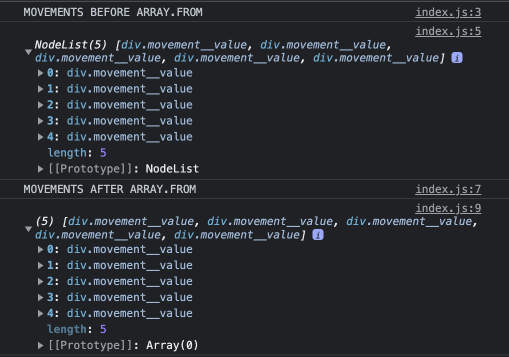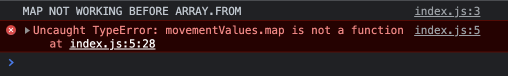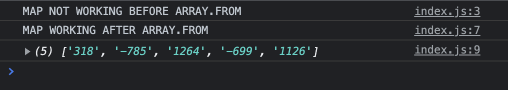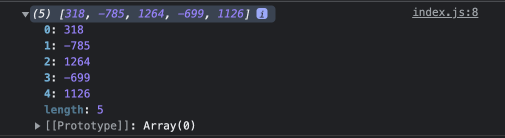# const - JS | lectureWorking With Arrays

Creating and Filling Arrays

In this lecture, we will learn how to create and fill arrays programmatically. So far, we have always created arrays like this.

script.js
``console.log( [9, 6, 3, 2, 1]);``

Or like this. Using the `new` keyword and the `Array` constructor.

script.js
``console.log( new Array(9, 6, 3, 2, 1));``

Now, in these cases, we basically already have all our data (9, 6, 3....). Hence we could then manually create these arrays. However, we can also generate arrays programmatically without having to define all the items manually.

There are many situations where this is necessary, and there are also multiple ways of doing it. And the easiest one is to use the `Array` constructor function again.

script.js
``const arr = new Array(5);``

You might think that the above line will create an array with only one element: 5. But that is not the case. It will create an array with 5 empty elements.

script.js
``````/**
* In some some browsers like Chrome, the output will be:
* [empty × 5]
*/
console.log(arr);``````Now, the array `arr` cannot be used for anything right now. We might want for example to use the map method on it and do something like this:

script.js
``````const arr = new Array(5);
console.log(arr.map(() => 8)); // [empty × 5]``````

But as you can see, the array is still empty. Nothing happens. There is however one method that we can call on this empty array. And that is the `fill` method. That's a new method we haven't studied yet but it's very straightforward.

Let me show it to you.

script.js
``arr.fill(8);``

When calling the fill method on an array, you need to pass in the value. That value will then be used to fill the array. Note that this method mutates the underlying array.

script.js
``console.log(arr); // [8, 8, 8, 8, 8]``

The `fill` method is a little bit similar to the `slice` method. In the `fill` method, besides the value that we want to fill the array with, we can also specify the index at which we want to start filling the array. So, let's say we want to start filling the array at index 2.

script.js
``console.log(arr.fill(8, 2)); // [ , , 8, 8, 8 ]``

As you can see it starts filling the array at index 2. It fills up until the end of the array unless we specify an end index just like with the `slice` method. Let's say index 4 is the end index.

script.js
``console.log(arr.fill(8, 2, 4)); // [ , , 8, 8,  ]``

Note that just like with the `slice` method, the end index is not included in the filling.

The `fill`method can also be use on already existing arrays. It doesn't have to be an empty array. Let's say we have an array of integers.

script.js
``const arr = [1, 2, 3, 4, 5];``

We can call the `fill` method on it and for example fill it with the value 12 from index 1 to index 4.

script.js
``console.log(arr.fill(12, 1, 4)); // [1, 12, 12, 12, 5]``

Next, let's say we have the below array.

script.js
``const arr = [1, 2, 3, 4, 5, 6, 7, 8, 9, 10];``

How could we create this array programmatically? Well, we could use the `Array.from` function.

script.js
``Array.from(/*...*/);``

As you can see, we are not using `from` as a method on an array. Instead, we are using it on the `Array` constructor. So, `Array` is a function, and on that function object, ce call the `from` method. Might sound a little bit confusing right now, but we will make more sense of it when we will talk about object-oriented programming.

Into the `from` function, we pass in as argument an array-like object, and a mapping function that will be called for each element.

``````const result = Array.from({ length: 10 }, (_, index) => index + 1);
console.log(result); // [1, 2, 3, 4, 5, 6, 7, 8, 9, 10]``````

Three things to note here. First, an array-like object is an object that has a non-negative length property and indexed elements. For example:

``````const arrayLike = {
0: 'a',
1: 'b',
2: 'c',
length: 3,
};``````

Secondly, the mapping function is exactly like the one in the map method. And finally, in the mapping function, the first parameter is the current value in the array but since we don't need it, I used an underscore as the first parameter which if you remember, represents a throwaway variable.

Let's now move on and see a more real use case of the `Array.from` function. Note that the `Array.from` function was initially introduced into JavaScript to create arrays from array-like structures.

Remember when we talked about Strings, Maps and Sets? They are all Iterables in JavaScript. Hence, they can be converted to real arrays using the `Array.from` function. And that's the reason also for the name of the function, because we can create arrays from other things.

Now, besides these obvious Iterables that I just mentioned, another great example of an array-like structure is the result of using `querySelectorAll`. In case you don't remember, `querySelectorAll` returns something called a NodeList, which is something like an array, which contains all the selected elements. It's not a real array. Hence, doesn't have methods like `map` and `reduce`.

So if we actually wanted to use a real array method like `map` or `reduce` on a NodeList, we would first need to convert the NodeList to an array. And for that `Array.from` is perfect.

Let's see it in action. Let's consider the below html code.

index.html
``````<body>
<div class="movement__value">318€</div>
<div class="movement__value">-785€</div>
<div class="movement__value">1264€</div>
<div class="movement__value">-699€</div>
<div class="movement__value">1126€</div>
</body>``````

Now let's say we want to get these values and calculate their sum. This means we need to somehow get them first from the user interface and then do the calculation based on that.

Let's get the values from the user interface.

script.js
``````console.log('MOVEMENTS BEFORE ARRAY.FROM');
const movementValues = document.querySelectorAll('.movement__value');
console.log(movementValues);

console.log('MOVEMENTS AFTER ARRAY.FROM');
const values = Array.from(movementValues);
console.log(values);``````Hope you can see the difference. Now let show you that we cannot call the `map` method on the `movementValues` array. Because the `map` method will be useful to get the numbers from the user interface, and also remove the euro symbol.

script.js
``````console.log('MAP NOT WORKING BEFORE ARRAY.FROM');
const movementValues = document.querySelectorAll('.movement__value');
console.log(movementValues.map((el) => el.textContent.replace('€', '')));

console.log('MAP WORKING AFTER ARRAY.FROM');
const values = Array.from(movementValues);
console.log(values.map((el) => el.textContent.replace('€', '')));``````Let's now keep on working only with `Array.from`. We can actually make our code even better by the second argument of the `Array.from` function. Which remember is the mapping callback function. And also, let's not forget to convert the values to numbers.

script.js
``````const values = Array.from(movementValues, (el) =>
Number(el.textContent.replace('€', ''))
);
console.log(values);``````All that's now left to do is to calculate the sum of all the values. You can do that as a challenge 😉 .

To finish this lecture, I just want to mention that another way of converting the NodeList to an array is by using the spread operator but then you will have to do the mapping separately.

script.js
``const values = [...movementValues]``